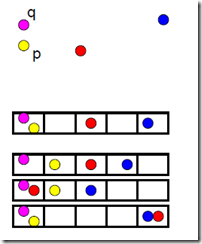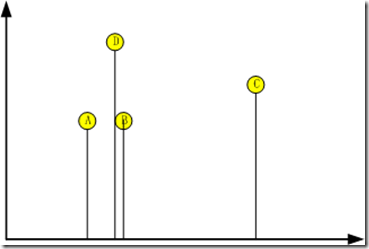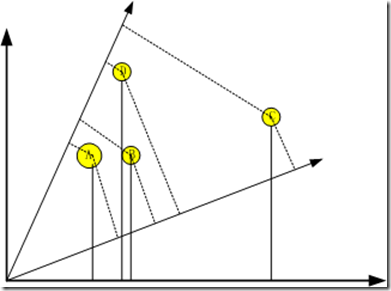# LSH那些事儿 (II): 图说

Author: Steven Date: Apr 18, 2013 Updated On: May 27, 2022
Categories: LSH那些事儿
Tags: Hash Index LSH
611 words in total, 2 minutes required.

### 1. 定义回顾

LSH旨在构造一种Hash函数集$\{ D \mid R^d \rightarrow U \}$，其中$d$是点的维数，使得对任意的点$\mathbf{p}$，$\mathbf{q}$有

1. 如果$|| \mathbf{p} - \mathbf{q} || \leq r$, 那么$\mathsf{Pr}(D(\mathbf{p}) = D(\mathbf{q}))$要很高
2. 如果$|| \mathbf{p} - \mathbf{q} || \geq cr$, 那么$\mathsf{Pr}(D(\mathbf{p}) = D(\mathbf{q}))$要很低### 2. 基于投影的LSH原理### 3. $(P_1,P_2,r,cr)$-sensitive LSH

A family $H$ of functions $D: R^d \rightarrow U$ is called $(P_1,P_2,r,cr)$-sensitive, if for any $\mathbf{p}$，$\mathbf{q}$:

1. if $|| \mathbf{p} - \mathbf{q} || \leq r$, then $\mathsf{Pr}(g(p) = g(q)) > P_1$
2. if $|| \mathbf{p} - \mathbf{q} || \geq cr$, then $\mathsf{Pr}(g(p) = g(q)) < P_2$继续阅读系列下一篇，将会对LSH的哈希家族进行阐述。